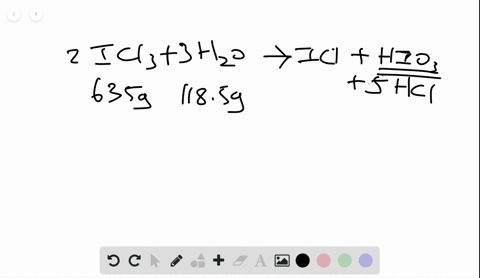🎉 The Study-to-Win Winning Ticket number has been announced! Go to your Tickets dashboard to see if you won! 🎉View Winning Ticket### Calculate the maximum numbers of moles and grams …

06:19University of Maine
Problem 79

# Metal hydrides react with water to form hydrogen gas and the metal hydroxide. For example,$$\mathrm{SrH}_{2}(s)+2 \mathrm{H}_{2} \mathrm{O}(l) \longrightarrow \mathrm{Sr}(\mathrm{OH})_{2}(s)+2 \mathrm{H}_{2}(g)$$You wish to calculate the mass (g) of hydrogen gas that can be prepared from 5.70 $\mathrm{g}$ of $\mathrm{SrH}_{2}$ and 4.75 $\mathrm{g}$ of $\mathrm{H}_{2} \mathrm{O}$ .(a) What amount (mol) of $\mathrm{H}_{2}$ can be produced from the givenmass of $\mathrm{SrH}_{2} ?$(b) What amount (mol) of $\mathrm{H}_{2}$ can be produced from the givenmass of $\mathrm{H}_{2} \mathrm{O}$ ?(c) Which is the limiting reactant?(d) How many grams of $\mathrm{H}_{2}$ can be produced?

## Discussion

You must be signed in to discuss.

## Video Transcript

in a chemical reaction. When you have quantities of both, reactant is given. You need to determine which is the limiting reactant. This is the one that determines the amount of product formed, so it's helpful to create a little road map to see how to go from given quantities to needed quantities. So if you have a mass of a substance, our first step is to change it. Two moles of that substance and we do that using the molar mass from the periodic table. Once we have moles of a given substance, we can change it to the moles of the substance we want. So in this case, we're wondering how much H two will form, and we can relate the two quantities using the ratio from the balanced equation or the coefficients. This is called the mole ratio from the balanced equation. So we take both of our quantities and we change them two moles and then we can convert them to moles of the product or moles of H two. Which ever quantity is smaller will be our limiting re agent. Let's start off with our strong iam hydride. We have five points 70 grams of strong iam hydride and the molar mass of strong um hydride is found by adding up the molar masses of each of the elements from the periodic table. So we look up the molar mass of Strong Liam, which is 87.62 plus the molar mass of hydrogen times, too, because there are two hydrogen two times 1.0 zero a. This tells us that one mole of striatum hydride has a mass of 89 0.636 grams. We also conceive from the balanced equation that every one mole of strong um hydride will produce two moles of hydrogen because those were the coefficients from the balanced equation. Now we have all the information we need to convert through a road map 5.70 grams of strong iam hydride, and we'll divide by the molar mass to give us moles. And then we multiplied by the ratio of two moles each two for every one mole of strong, um, hydride to give us 0.1 to 7 moles of hydrogen produced for the same type of calculation. With the amount of water that we start off with, we have 4.75 grams of H 20 the molar mass, using the individual molar masses of the elements physical to 18.15 grams. And I also know that for every two moles of water, two moles of hydrogen will be produced. But I convert 4.75 grams. It's 202 moles, h 20 and then for moles of H 202 Moles of age to this gives 0.264 moles of age to so because 0.1 to 7 is less than 70.264 That indicates that strong IAM hydride is the limiting re agent. And we can determine the mass hydrogen that will be produced by using the amount of mold produced by the limiting re agent. So if we have 0.1 to 7 moles of hydrogen, no, we want to find out how many grams at equals. We use the molar mass of hydrogen when we multiply, so 0.1 to 7 moles times everyone mole has a mass of two times 1.8 grams or point 256 grams of hydrogen is produced